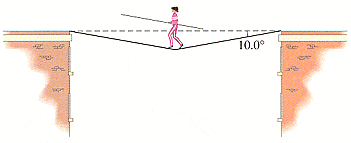# Help figuring out Tension Force

Damie904
1.Homework StatementArlene is to walk across a high wire strung horizontally between two buildings 13.0 m apart. The sag in the rope when she is at the midpoint is 10.0°. If her mass is 50.0 kg, what is the tension in the rope at this point?
So I know:
Mass=50kg
Acceleration=0 m/s2

## Homework Equations

This is the part I need help with because I'm not exactly sure what formula to use to find tension motion. Since F=ma would just give me 0 which would be incorrect.
The only other Formula I can think of is 2cos(theta)Ty=mg, but I'm not too sure if that's correct. Plus, I don't know what Ty is.

Last edited:

## Answers and Replies

Pi-Bond
Resolving the tension along the vertical axis should not give $2cos(\theta)T_{y}=mg$. What do you think it should?

Fewmet
You are sort of correct that the F=ma=0. Really, it is that ∑F=0, where ∑F is the sum of all the forces acting on Arlene. I think you see that is her weight pulling down, but what is pushing her up? Something has to be if ∑F=0.

Consider that the point under her feet is being pulled left by one part of the rope and right by the other part of the rope. Those forces are ±Tx.

Each of the two parts of the rope are also pulling her up. The upward force of one part would be Ty.

Do you see why there would be 2Ty? And why that would equal mg?

(Check your notes or your text: I think you'll see that you've mistranscribed 2cos(theta)Ty=mg)